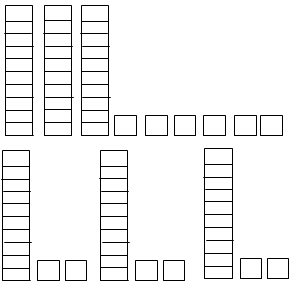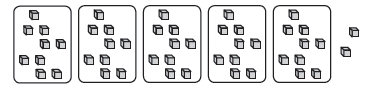# Lesson Notes By Weeks and Term - Primary 3

Division

TERM: 3rd Term

WEEK: 2

CLASS: Primary 3

AGE: 8 years

DURATION: 5 periods of 40 minutes each

DATE:

SUBJECT: Mathematics

TOPIC: Division

SPECIFIC OBJECTIVES: At the end of the lesson, the pupils should be able to

1. Divide numbers to 99 by 1, 2, 3, 4, 5, 10.
2. Solve word problems in context and explain own solutions to problems that involve equal sharing and grouping up to 100 with answers that can include remainders.
3. Use appropriate symbols ÷, =, +, â¡.

INSTRUCTIONAL TECHNIQUES: Explanation, question and answer, demonstration, practical, assessments

INSTRUCTIONAL MATERIALS: Base ten blocks, Whiteboards/scrap paper, videos from source-

PERIOD 1: Division- grouping and sharing

 PRESENTATION TEACHER’S ACTIVITY PUPIL’S ACTIVITY STEP 1MENTAL MATHS The teacher begins the lesson with some mental calculationsCalculateWhat is 100 more than1. 814 2. 206 3. 54  4. 154 5. 754  6. 8767. 8678. 7869. 76810. 687 Pupils respond and participate STEP 2CONCEPTDEVELOPMENT The teacher• Revise breaking down numbers into tens and units with the learners.• For example:13 = 10 + 3               68 = 60 + 824 = 20 + 4               72 = 70 + 235 = 30 + 5               84 = 80 + 446 = 40 + 6               93 = 90 + 357 = 50 + 7               14 = 10 + 4.  CLASS ACTIVITYThe teacher• Does the following examples on the board:−− Share 24 between 2. Use base ten blocks to demonstrate the sharing and record the numeric workingwhile you explain what you are doing.24 ÷ 2 =___−− Share 39 among 3. (Use blocks to demonstrate and talk about the steps in the working while you do the calculation.)39 ÷ 3 = ___= (30 + 9) ÷ 3= (30 ÷ 3) + (9 ÷ 3)= 10 + 3= 13 ACTIVITY IIThe teacher• Asks learners to do the following examples on their whiteboards/scrap paper. Use the method used above.−− Share 48 among 4. Use base ten blocks to demonstrate the sharing.−− Share 28 between 2. Use base ten blocks to demonstrate the sharing. Pupils pay attention and participate STEP 3CLASS-WORK 1. Write in expanded notation.a) 19 = ___ + ___b) 41 = ___ + ___c) 24 = ___ + ___d) 58 = ___ + ___e) 63 = ___ + ___f) 82 = ___ + ___g) 76 = ___ + ___h) 94 = ___ + ___ 2. Write the numbers in expanded notation before dividing.a) 39 ÷ 3 = ___b) 45 ÷ 5 = ___ 3. Max makes 50 cakes. He puts them in bags with 5 cakes per bag. How many bags can he make? 4. Grant makes small bags of gums to sell at school. He has a big bag with 80 gums.He puts 4 gums in a bag. How many small bags can he make? Pupils attempt their class work STEP 4HOME-WORK 1. Write in expanded notation.a) 23 = ___ + ___b) 86 = ___ + ___2. Divide the following by writing the numbers in expanded notation first:a) 48 ÷ 4 = ___b) 55 ÷ 5 = ___ Pupils attempt their class work STEP 5SUMMARY The teacher summarizes by reminding the pupils how to divide by sharing and grouping She marks their class works, makes corrections where necessary and commends them positively

PERIOD 2 : Division-revise sharing

 PRESENTATION TEACHER’S ACTIVITY PUPIL’S ACTIVITY STEP 1MENTAL MATHS The teacher begins the lesson with some mental calculationsWhat is 100 less than1. 376 2. 768 3. 321 4. 453 5. 567  6. 802  7. 9718. 4539. 19910. 567 Pupils respond and participate STEP 2CONCEPTDEVELOPMENT The teacher• Revises the distributive property with the learners.• Does the following examples using base 10 blocks.Share the tens and then the units.Example with no remainder:36 ÷ 3 = â¡47 ÷ 5 = â¡• Asks how many units are left (if any). That will be a remainder (we write it rem).In the first example there is no remainder. So 36 ÷ 3 = 1 ten and 2 units = 12In the second example the remainder is 2. So 47 ÷ 5 = 9 rem 2 (You need to exchange 1 ten for 10 units to do this sharing.)  CLASS ACTIVITYThe teacher• Explains to learners that we can also show the same calculations on the board as follows.• Explains the use of the brackets when multiplying out using the distributive law. You do not have to use the term distributive law, but learners do need to understand the working and know how to write it out correctly.This method works when the broken up number can be divided completely by the divisor.(30 + 7) ÷ 3                         (40 + 7) ÷ 5= (30 ÷ 3) + (7 ÷ 3)           = (40 ÷ 5) + (7 ÷ 5)= (10 + 2) rem 1               = (8 + 1) rem 2= 12 rem 1                        = 9 rem 2• Asks learners to do the following examples on their whiteboards/scrap paper. They must hold up theirwhiteboards/scrap paper after completing each example for you to check before proceeding with the next example.−− 25 ÷ 5 = ___ (5)−− 25 ÷ 4 = ___ (6 rem 1)−− 25 ÷ 2 = ___ (12 rem 1)−− 25 ÷ 10 = ___ (2 rem 5)−− 25 ÷ 3 = ___ (8 rem 1) Pupils pay attention and participate STEP 3CLASS-WORK 1. Share 14 sweets among 3 children:a) How many sweets do they each get?b) How many sweets are left over? 2. Share 13 sweets among 5 children. How many sweets each? How many left over? 3. Share 19 sweets among 5 children. How many sweets each? How many left over? 4. Write the numbers in expanded notation before dividing.a) Share 30 marbles among 4 children. How many marbles are left? b) Share 19 marbles between 2 children. How many marbles are left? 5. Calculate the following:a) 25 ÷ 5 = ___b) 63 ÷ 5 = ___ Pupils attempt their class work STEP 4HOME-WORK Divide the following by writing the numbers in expanded notation first:a) Share 47 marbles among 5 children. How many marbles are left? b) Share 29 marbles among 4 children. How many marbles are left? Pupils attempt their class work STEP 5SUMMARY The teacher summarizes by reminding the pupils how to divide numbers She marks their class works, makes corrections where necessary and commends them positively

PERIOD 3: Division-word problems

 PRESENTATION TEACHER’S ACTIVITY PUPIL’S ACTIVITY STEP 1MENTAL MATHS The teacher begins the lesson with some mental calculationsCalculateWhat is 200 more than1. 376 2. 768 3. 321 4. 4535. 567 6. 265  7. 763  8. 289. 70610. 219 Pupils respond and participate STEP 2CONCEPTDEVELOPMENT The teacher• Does the following problems with the learners.The farmer wants to sell apples. He sells them in bags of 3 apples. He has 66 apples. How many bags of apples can he make up?• Uses the layout shown below to illustrate on the board how you have shared the apples.How can we write this as a division number sentence? (66 ÷ 3 = 22)CLASS ACTIVITYThe teacher• Does another problem-solving example with the learners.Three teachers share 98 books so that they each get the same number of books for their classes. How many books does each teacher get for her class? • Asks the learners to draw three circles (one for each teacher) on their whiteboards/scrap paper and to share the books between the circles. They should begin by thinking about all the big number facts they remember about their 3x tables. You should write out the working on the board (similarly to example in Activity 1 above). There may be different ways of sharing the books. Discuss various suggestions. E.g.30 + 30 + 30 + 8 = 98. Each gets 30 books and they share the last 8 books. Each gets 2 of those books, so they each get 32 books and there are 2 books left over.How can we write this as a division number sentence? (98 ÷ 3 = 32 rem 2) ACTIVITY IIThe teacher• Works through another example – allow time for learners to work on their whiteboards/scrap paper before discussing the solution with the class.Mum divides 62 eggs to use equally over 5 days. How many eggs does she have for each day? (12 rem 2) Pupils pay attention and participate STEP 3CLASS-WORK Draw a picture and write a division number sentence and answer for these problems:1. The baker wants to sell bread rolls. He sells them in bags of 6 each.He has 56 rolls. How many bags of rolls can he make up? 2. Four children share 86 sweets so that they all get the same number of sweets.How many sweets does each child get? 3. Kelvin has 58 marbles. He wants to put them in bags of 5 each to give to his friends.How many bags of 5 marbles each can he make up? Pupils attempt their class work STEP 4HOME-WORK Solve the problem, by drawing circles and then write a number sentence:1. Four sisters want to share N63 so that they all get the same amount of money in naira.How many naira will each sister get? 2. Six boys want to share 25 toy cars so that they all get the same number of toy cars to play with. Pupils attempt their class work STEP 5SUMMARY The teacher summarizes by reminding the pupils how to solve word problems involving division She marks their class works, makes corrections where necessary and commends them positively

PERIOD 4: Multiplication and division- inverse operations

PERIOD 5: Weekly Test/consolidations

TEACHER’S ACTIVITY: The teacher revises all the concepts treated from period 1-4 and gives the pupils follow through exercises, quiz and tests. She marks the exercises, makes corrections and commends the pupils positively.

PUPIL’S ACTIVITY: The pupils work on the worksheets and exercises given by the teacher individually

CONSOLIDATION

Calculate the following. Use any method that you have learned in class. Show your method.

1. 44 ÷ 4 =
2. 84 ÷ 4 =
3. 28 ÷ 2 =
4. 40 ÷ 2 =
5. 65 ÷ 5 =
6. 90 ÷ 5 =
7. 20 ÷ 2 =
8. 64 ÷ 4 =
9. 55 ÷ 5 =
10. 28 ÷ 4 = ___
11. 78 ÷ 2 = ___
12. 90 ÷ 2 = ___

1. The vendor has 63 tomatoes. He wants to sell them in packets of 3 each.

How many packets of tomatoes will he be able to make up?

1. I have 55 silk worms. I want to share them between myself and my four friends.

How many worms will we each get?

1. My brother has 44 toy cars. He wants to share them among himself and his 3 friends

when they come over to play. How many cars will they each get to play with?